# 9.1.3. Inverting Corrected Gravity Data¶

## 9.1.3.1. Prelude¶

Here, we show how GIFtools can be used to invert gravity anomaly data. We consider the case where we have a set of field observations and some a priori knowledge of the local geology; for this example, we know the anomaly is produced by the TKC kimberlites. We assume that all necessary corrections have been applied to the raw gravity data; see the processing gravity data exercise. The goal of this exercise is to invert the gravity anomaly data to recover the optimum density model. Several inversion will be run to show the impact of reference models and various penalty terms on the final inversion result.

Tip

The same workflow can be used to invert magnetic data for an arbitrary susceptibility or magnetic vector model.

## 9.1.3.2. Setup for the Inversion Exercise¶

If you have completed the tutorial “Forward Model Gravity Data and Compare Against Field Observations”:

If you have NOT completed the previous tutorial, you must complete the following steps:

Tip

• Requires at least GIFtools version 2.1.3 (Oct 2017) (login required)

## 9.1.3.3. Assign Uncertainties and Set I/O Headers¶

Assigning appropriate uncertainties to the data is necessary for running stable and successful inversions with GIFtools. Because the observed gravity data were generated synthetically, we will add random noise before assigning the uncertainties. Because the statistics of the noise are known, they can be used to assign the correct uncertainties. Complete the following steps: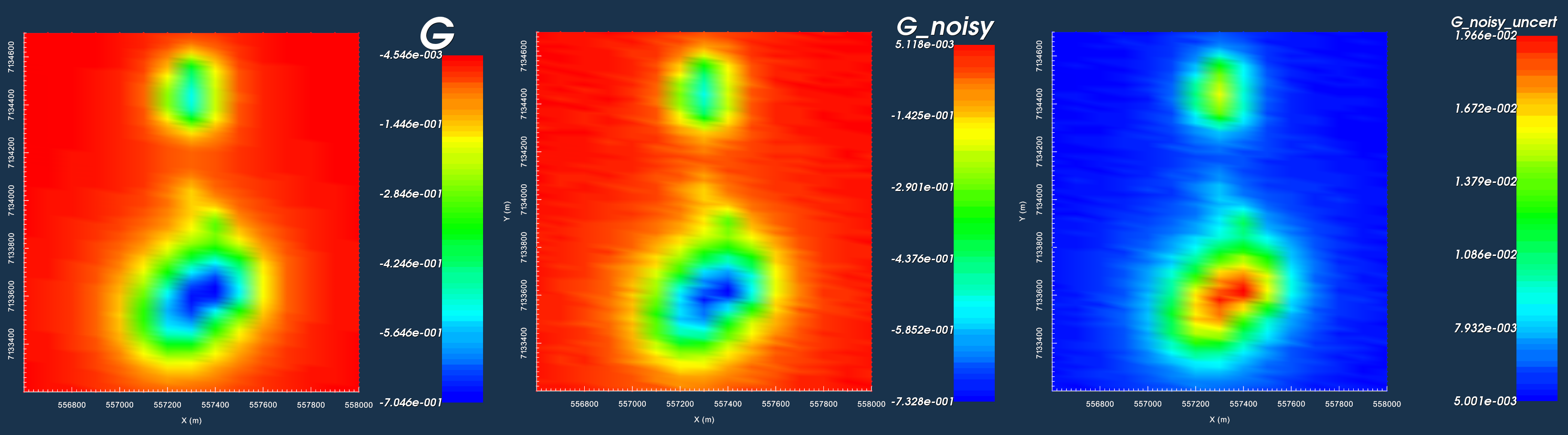Synthetic gravity anomaly data (left). Gravity anomaly data with noise (middle). Uncertainties on noisy data (right).

## 9.1.3.4. Unconstrained Smooth Inversion¶

Here, we perform the most basic type of gravity anomaly inversion. No a priori information is used in the inversion. Default inversion parameters use least-squares penalties on the model and its gradients. As a result, we expect the inversion to recover a smooth model. To run the inversion and view results:

Note

The user is encouraged to:

• Compare predicted and observed data and examine the misfit

• Lay the observed data over the final model to see if observed anomalies match the distribution of recovered densities

• Lay the geological image over the final model to see if the inversion results agree with geological surface mapping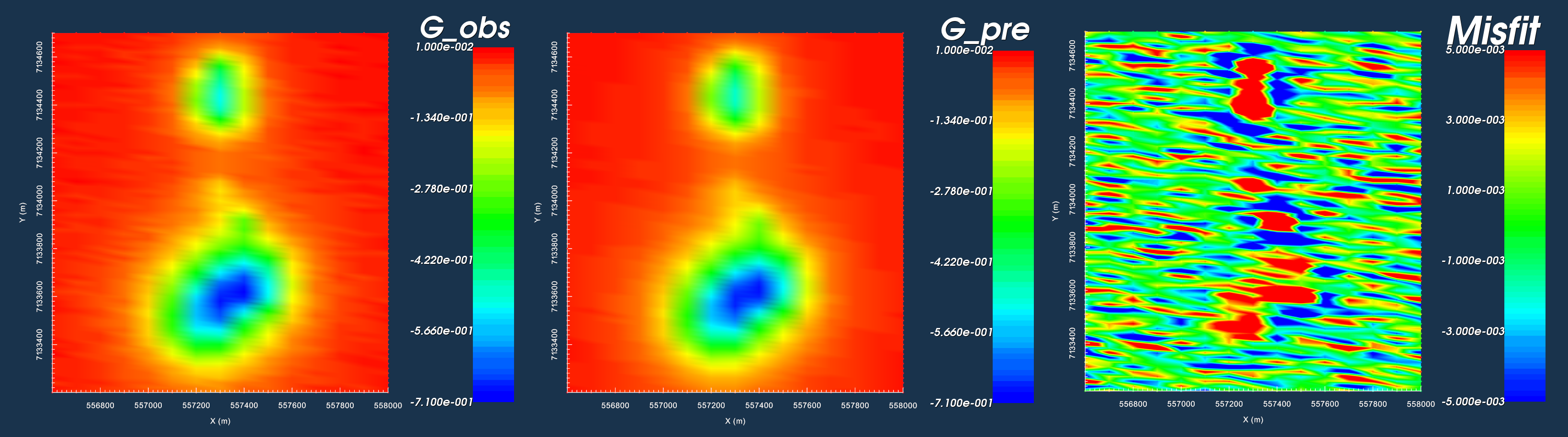Observed data (left). Data predicted with final model (middle). Misfit with colour scale set to +/- 0.005 (right).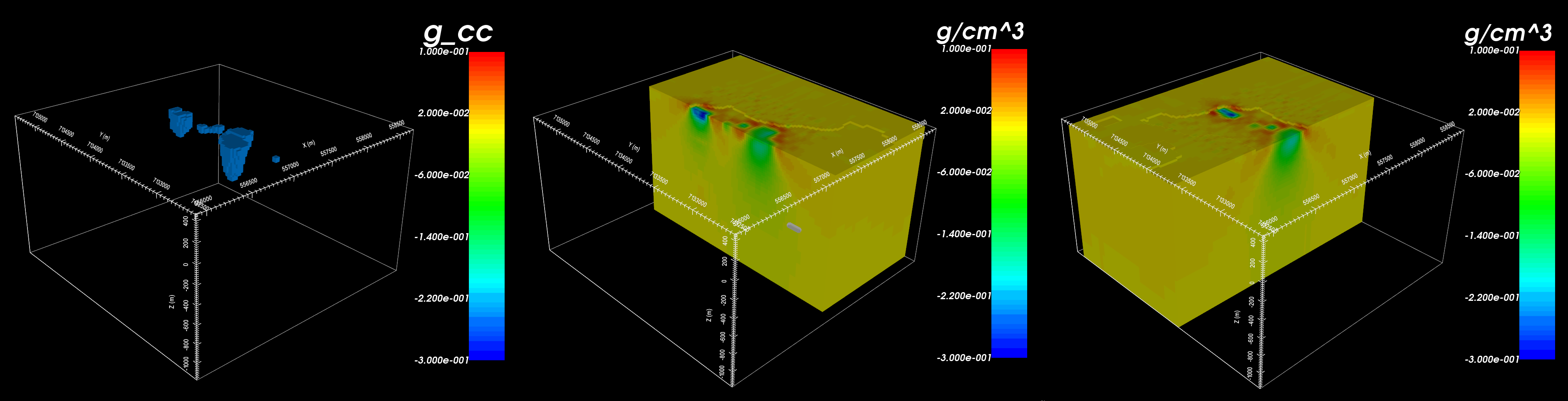True model with only non-zero density contrasts (left). NW slice of recovered model (middle). EW slice through southern anomaly (right).

### 9.1.3.4.1. Results¶

• Generally, the data predicted using the recovered model matches the shape and character of the observed anomalies

• However, large misfits are clustered around the locations of recovered structures

• The general distribution of density contrasts is recovered through inversion

• By using the default set of inversion parameters however, we recovered a very smooth density contrast model

• Because the inversion was set to recover a smooth model, the inversion placed positive density contrast values (red) around the outside of the recovered structures

## 9.1.3.5. Inversion with Hard Constraints¶

Here, we show the impact of reference models on the final inversion result. Two inversion will be run - one using SMOOTH_MOD and one using SMOOTH_MOD_DIFF. Both inversions are constrained with the model that was made using the geological surface map (see here). To complete this exercise:

Note

The user is encouraged to:

• Examine the differences in recovered models using SMOOTH_MOD and SMOOTH_MOD_DIF

• Examine the differences in data misfit for data predicted using SMOOTH_MOD and SMOOTH_MOD_DIF inversions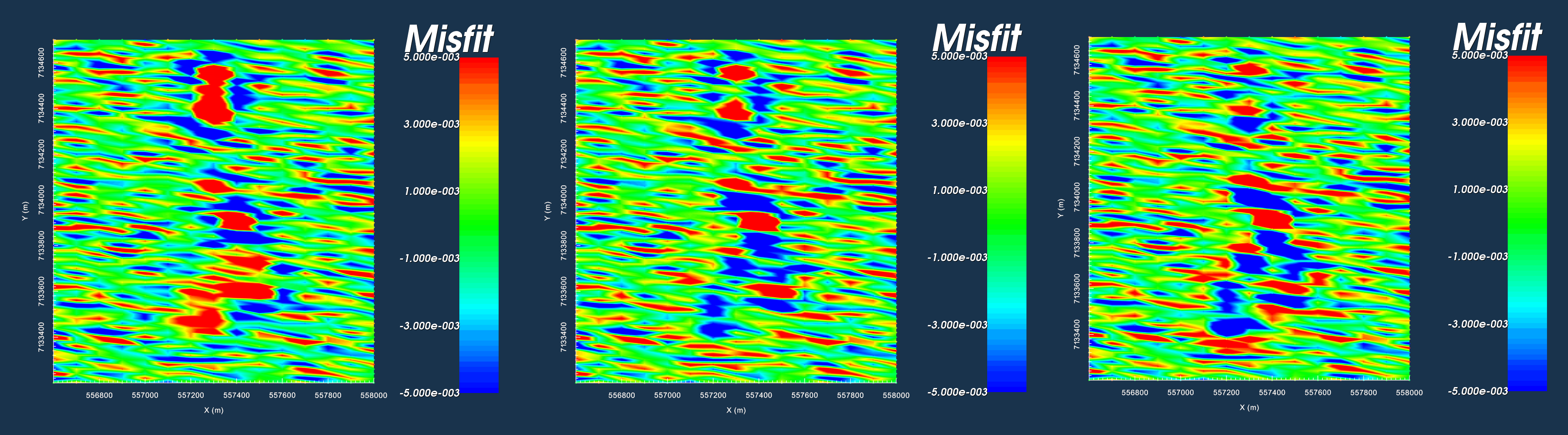Data misfit thresholded at +/- 0.005 for the unconstrained smooth model (left), SMOOTH_MOD (middle) and SMOOTH_MOD_DIF (right).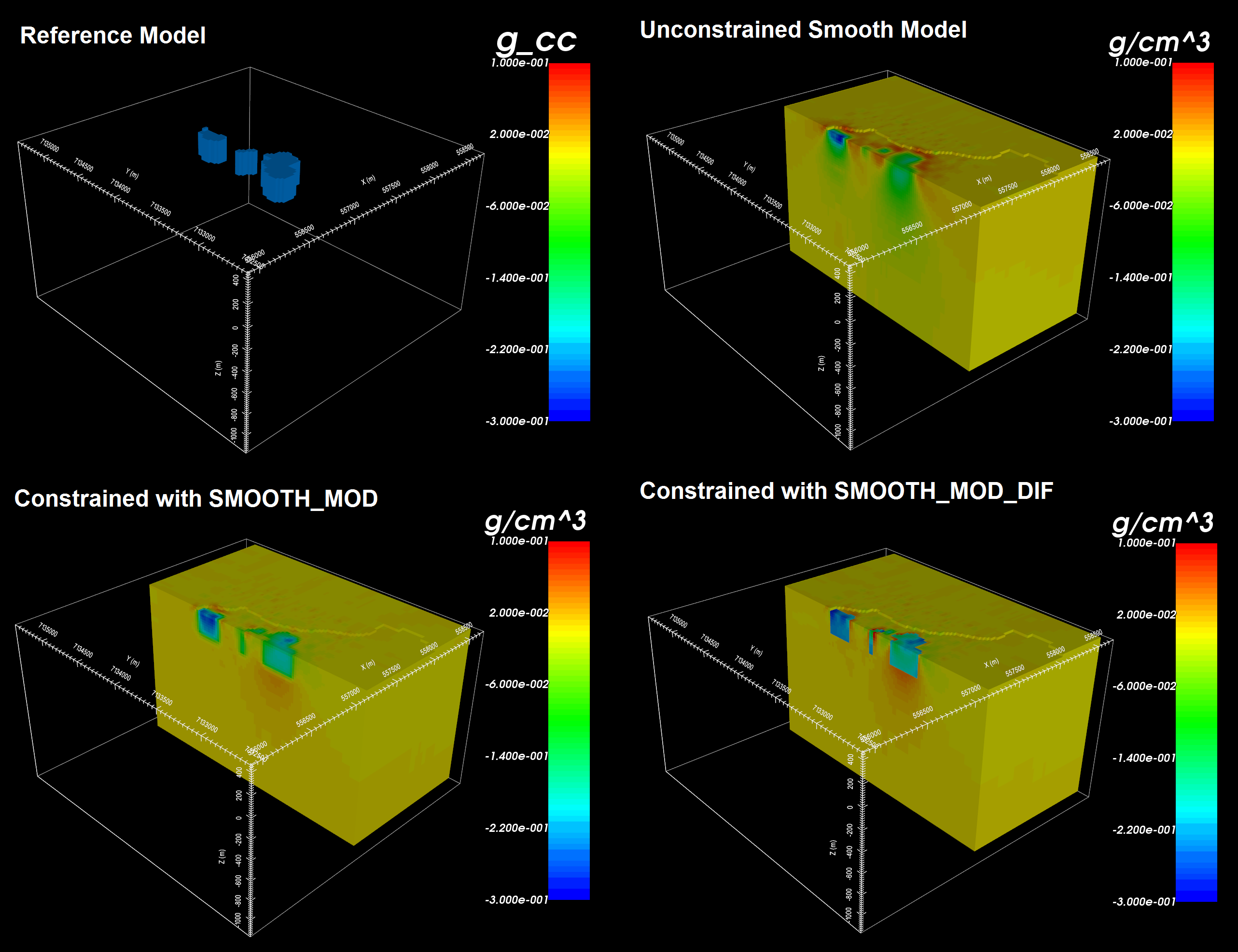Reference model from geological unit image (top-left). Model recovered using unconstrained smooth inversion (top-right). Model recovered using SMOOTH_MOD (bottom-left). Model recovered using SMOOTH_MOD_DIF (bottom-right).

### 9.1.3.5.1. Results¶

• All three inversion match the data fairly well but with some anomalously high misfits over the location of the kimberlites.

• SMOOTH_MOD imposes the structures of the reference model but allows smooth gradients

• SMOOTH_MOD_DIFF imposes the structures and gradients of the reference model

## 9.1.3.6. Inversion with Soft Constraints¶

Here, we show how compact and blocky models can be recovered by changing certain inversion parameters. Smooth models are recovered when the compactness parameter (p) and the set of blockiness parameters (q) are set to a value of 2. By decreasing the compactness parameter value, we recover models that have a smaller number of non-zero values; that is, models which fit the observed data using more compact structures. By decreasing the blockiness parameter value, we recover models that have a smaller number of non-zero gradients; that is, models which fit the observed data using structures that have very sharp edges. To complete this exercise:

Note

The user is encouraged to:

• see how changes in the compactness parameter (p) affect recovered models

• see how changes in the blockiness parameters (q) affect recovered models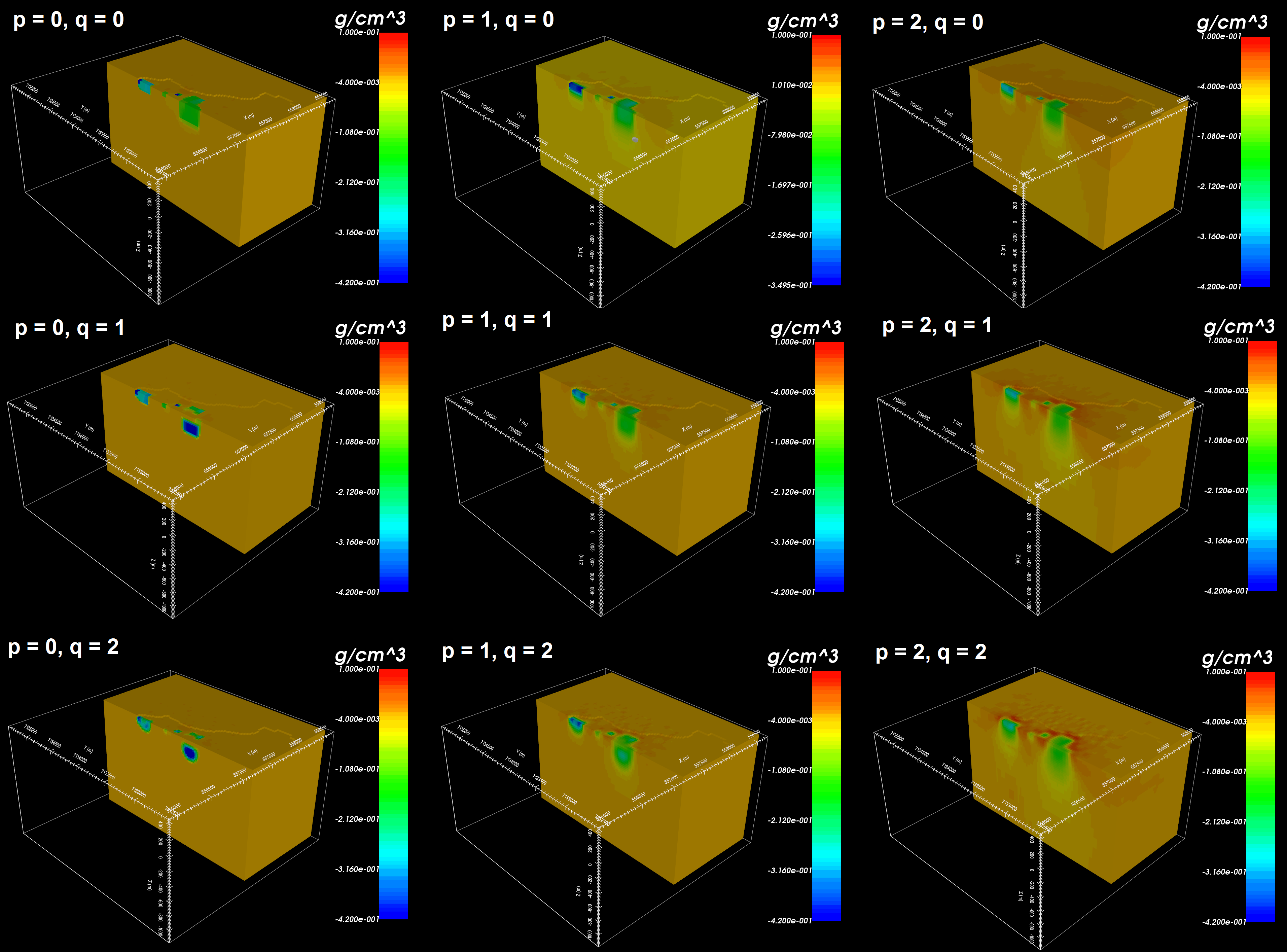Inversion results for various soft constraints.